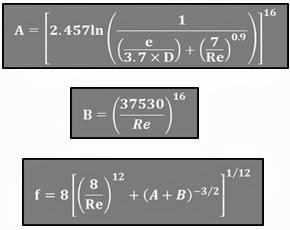# Friction Factor Function

August 3, 2011

Last updated: 30/09/2017, 1 min read### Introduction

Friction factor once again. This time I have developed a custom VBA function that calculates the friction factor based on Churchill’s equation. If you have read my previous post, you will probably remember that Churchill’s equation is valid for the entire Reynolds range (any type of flow). The equation involves the calculation of two intermediate values that are used in the final equation. This equation is also included on ASHRAE’s Fundamentals.### VBA code

The code for this function is given below:

`Option Explicit Function FrictionFactor(Roughness As Double, Diameter As Double, Velocity As Double, Viscosity As Double)        '-----------------------------------------------------------------------------    'Calculates the friction factor of a pipe using Churchill's equation (1977).    'This equation is valid for all types of flows (from laminar to turbulent).        'Written Βy:    Christos Samaras    'Date:          04/08/2011    'E-mail:        [email protected]    'Site:          https://myengineeringworld.net/////    '-----------------------------------------------------------------------------        'Declaring the necessary variables.    Dim Reynolds    As Double    Dim A           As Double    Dim B           As Double        'Calculate the Reynolds number.    Reynolds = (Diameter * Velocity) / Viscosity        'Calculate the intermediate variables A and B.    A = ((2.457 * WorksheetFunction.Ln(1 / ((Roughness / (3.7 * Diameter)) + ((7 / Reynolds) ^ 0.9)))) ^ 16)    B = (37530 / Reynolds) ^ 16        'Apply the equation.    FrictionFactor = 8 * ((((8 / Reynolds) ^ 12) + ((A + B) ^ (-3 / 2))) ^ (1 / 12))End Function`

The above function can be found in the file below. The function can be used as the regular built-in functions of Excel. Fill the necessary arguments and the function will calculate the friction factor. Just note to fill the values with the correct units.

The file can be opened with Excel 2007 or newer. Note that you should enable macros to use it.

Category: Office Tips